# Series "changers"

These commands change the original series:

###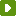clear

Deletes all elements from the series.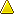Simply assigning "" (empty string) or zero to a series may not produce the expected results. Red's logic makes it seem to "remember" things in unexpected ways. To really clear it, use `clear`.

``````>> a: [11 22 33 "cat"]
== [11 22 33 "cat"]
>> clear a
== []
>> a
== []
``````

###poke

Changes the value of a serie's element at the position given by the second argument to the value of the third argument.``````>> x: ["cat" "dog" "mouse" "fly"]
== ["cat" "dog" "mouse" "fly"]

>> poke x 3 "BULL"
== "BULL"

>> x
== ["cat" "dog" "BULL" "fly"]
``````
``````>> s: "abcdefghijklmn"
== "abcdefghijklmn"

>> poke s 4 #"W"
== #"W"

>> s
== "abcWefghijklmn"
``````

###append

Inserts the values of the second argument at the end of a series. Changes only the original first series.``````>>  x: ["cat" "dog" "mouse" "fly"]
== ["cat" "dog" "mouse" "fly"]

>> append x "HOUSE"
== ["cat" "dog" "mouse" "fly" "HOUSE"]

>> x
== ["cat" "dog" "mouse" "fly" "HOUSE"]
``````
``````>>  x: ["cat" "dog" "mouse" "fly"]
== ["cat" "dog" "mouse" "fly"]

>> y: ["Sky" "Bull"]
== ["Sky" "Bull"]

>> append x y
== ["cat" "dog" "mouse" "fly" "Sky" "Bull"]
>> x
== ["cat" "dog" "mouse" "fly" "Sky" "Bull"]
``````
``````>> append "abcd" "EFGH"
== "abcdEFGH"
``````

#### append/part

Limits the number of elements appended to the series.

``````>> append/part ["a" "b" "c"] ["A" "B" "C" "D" "E"] 2
== ["a" "b" "c" "A" "B"]
``````

#### append/only

Appends series A with series B, but B goes in as a series (block).

``````>> append/only ["a" "b" "c"] ["A" "B"]
== ["a" "b" "c" ["A" "B"]]
``````

#### append/dup

Appends series A with series B a given number of times. I think it should not be called dup from "duplicate" as it can triplicate, quadrupicate...

``````>> append/dup ["a" "b" "c"] ["A" "B"] 3
== ["a" "b" "c" "A" "B" "A" "B" "A" "B"]
``````

###insert

It is like `append`, but the addition is done at the current entry index (usually the beginning).While `append` returns the series from head, `insert` returns it after the insertion. This allows to chain multiple `insert` operations, or help calculate the length of the inserted part, but `a: insert a something` will not change "a"!``````>> a: "abcdefgh"
== "abcdefgh"
>> insert a "OOO"
== "abcdefgh"
>> a
== "OOOabcdefgh"
``````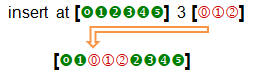``````>> a: "abcdefgh"
== "abcdefgh"
>> insert at a 3 "OOO"
== "cdefgh"
>> a
== "abOOOcdefgh"
``````

#### insert/part

Inserts only a given number of elements from the second argument.

#### insert/only

Allows insertion of blocks as blocks, not their elements.

#### insert/dup

Allows the insertion to be repeated a given number of times.

``````>> a: "abcdefg"
== "abcdefg"
>> insert/dup a "XYZ" 3
== "abcdefg"
>> a
== "XYZXYZXYZabcdefg"
``````

###replace

Replaces an element of the series.``````>> replace ["cat" "dog" "mouse" "fly" "Sky" "Bull"] "mouse" "HORSE"
== ["cat" "dog" "HORSE" "fly" "Sky" "Bull"]
``````
##### replace/all

replaces all ocurrences.

``````>> a: "my nono house nono is nono nice"
== "my nono house nono is nono nice"
>> replace/all a "nono " ""
== "my house is nice"
``````

###sort

Sorts a series.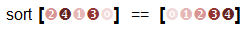``````>> sort [8 4 3 9 0 1 5 2 7 6]
== [0 1 2 3 4 5 6 7 8 9]
``````
``````>> sort "sorting strings is useless"
== "   eeggiiilnnorrsssssssttu"
``````

###remove

Removes the first value of the series.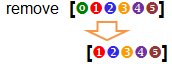``````>> s: ["cat" "dog" "fox" "cow" "fly" "ant" "bee"]
== ["cat" "dog" "fox" "cow" "fly" "ant" "bee"]
>> remove s
== ["dog" "fox" "cow" "fly" "ant" "bee"]
>> s
== ["dog" "fox" "cow" "fly" "ant" "bee"]
``````

#### remove/part

Removes a given number of elements``````>> s: "abcdefghij"
== "abcdefghij"
>> remove/part s 4
== "efghij"
``````

Notice that you can do the same with `remove at [0 1 2 3 4 5] 2` .

###take

Removes the FIRST element of a series and gives it as return.

``````>> s: ["cat" "dog" "fox" "cow" "fly" "ant" "bee"]
== ["cat" "dog" "fox" "cow" "fly" "ant" "bee"]
>> take s
== "cat"
>> s
== ["dog" "fox" "cow" "fly" "ant" "bee"]
``````
##### take/last

Removes the LAST element of a series and gives it as return.

``````>> s: ["cat" "dog" "fox" "cow" "fly" "ant" "bee"]
== ["cat" "dog" "fox" "cow" "fly" "ant" "bee"]
>> take/last s
== "bee"
>> s
== ["cat" "dog" "fox" "cow" "fly" "ant"]
```````take/last` and `append` can be used to perform stack (queue) operations.

##### take/part

Removes a given number of elements from the start of the series and gives them as return.

``````>> s: ["cat" "dog" "fox" "cow" "fly" "ant" "bee"]
== ["cat" "dog" "fox" "cow" "fly" "ant" "bee"]
>> take/part s 3
== ["cat" "dog" "fox"]
>> s
== ["cow" "fly" "ant" "bee"]
``````

###move

Moves one or more elements from the second argument into the first argument. Changes both original arguments.

.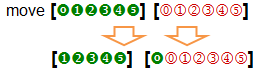To move more than one element, use the refinement `/part`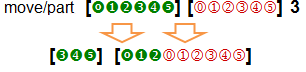``````>> a: [a b c d]
== [a b c d]
>> b: [1 2 3 4]
== [1 2 3 4]
>> move a b
== [b c d]
>> a
== [b c d]
>> b
== [a 1 2 3 4]
>> move/part a b 2
== [d]
>> a
== [d]
>> b
== [b c a 1 2 3 4]
>>
``````

`move` can be used combined with other commands to move things inside a single series. For example:

``````>> a: [1 2 3 4 5]
== [1 2 3 4 5]
>> move a tail a
== [2 3 4 5 1]
>> move/part a tail a 3
== [5 1 2 3 4]
``````

###change

Changes the first elements of a series and returns the series after the change. Modifies the first original series, not the second.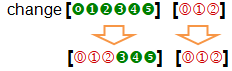``````>> a: [1 2 3 4 5]
== [1 2 3 4 5]
>> change a [a b]
== [3 4 5]
>> a
== [a b 3 4 5]
``````

Refinements:

/part - limits the amount to change to a given length.
/only - changes a series as a series.
/dup - duplicates the change a specified number of times

###alter

Either appends or removes an element from a series. If `alter` does NOT find the element in a series, it appends it and returns `true`. If it finds the element, removes it and returns `false`.

``````>> a: ["cat" "dog" "fly" "bat" "owl"]
== ["cat" "dog" "fly" "bat" "owl"]
>> alter a "dog"
== false
>> a
== ["cat" "fly" "bat" "owl"]
>> alter a "HOUSE"
== true
>> a
== ["cat" "fly" "bat" "owl" "HOUSE"]
``````

###swap

Swaps the first elements of two series. Returns the first series, but changes both: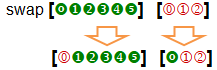``````>> a: [1 2 3 4] b: [a b c d]
== [a b c d]
>> swap a b
== [a 2 3 4]
>> a
== [a 2 3 4]
>> b
== [1 b c d]
``````

With `find` , for example, it can be used to swap any element of two series and even elements within a single series:

``````>> a: [1 2 3 4 5] b: ["dog" "bat" "owl" "rat"]
== ["dog" "bat" "owl" "rat"]
>> swap find a 3 find b "owl"
== ["owl" 4 5]
>> a
== [1 2 "owl" 4 5]
>> b
== ["dog" "bat" 3 "rat"]
``````

###reverse

Reverses the order of the elements of a series:

``````>> reverse [1 2 3]
== [3 2 1]
>> reverse "abcde"
== "edcba"
``````

Allows /part refinement to limit the reverse to the number of elements of the argument:

``````>> reverse/part "abcdefghi" 4
== "dcbaefghi"
``````